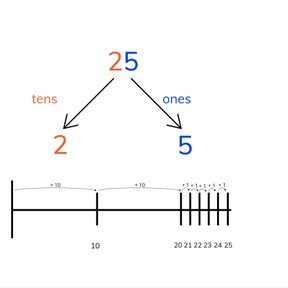Place value- numbers to 30 on the number line

# Place value- numbers to 30 on the number line

Place value- numbers to 30 on the number line

No account needed.8,000 schools use Gynzy92,000 teachers use Gynzy1,600,000 students use Gynzy

## General

Students start to learn about place value and learn to place numbers on the number line. They learn the tens and ones that make up numbers to 30 and can jump to these numbers on the number line.

1.NBT.B.2

## Relevance

Discuss with students that it is useful to know how numbers to 30 are put together so you can work with them later.

## Introduction

Teacher starts with a ball and says the number 1. Throw the ball to a student and have them say the next number, 2. They throw to another student who has to say, 3. Continue throwing and counting until you reach 30.

## Development

Show the tens and ones on the interactive whiteboard. Explain that tens are a group of 10 and ones are the numbers from 1 to 9. Show the MAB-blocks on the interactive whiteboard and/or show them in your classroom as well. Using the blocks, repeat the explanation of tens and ones. Show the TO-chart. Explain that the number 25 is made of 2 tens and 5 ones, and show how these jumps can be made on the number line. Point this out on the numbers next to the TO-chart. Show that you can also jump to 25 in a faster way by making jumps of 5 instead of jumps of 1. Practice as a class having students determine the tens and ones of a number. Discuss how to best jump to the number 29. Write the intermediate jumps on the interactive whiteboard. Next a few exercises are given in which students have to determine which number has been jumped to based on the given jumps on the number line.

Check that students know how to build numbers to 30 and place them on a number line by asking the following questions:
- What are tens? How do you know?
- What are ones? How do you know?
- Which jumps would you make on the number line to reach a given number?

## Closing

Discuss with students the importance of knowing how numbers to 30 are put together is, namely that you need this knowledge to do calculations later on. Check that students know what tens and ones are, and that they know how to jump to a given number on the number line. To practice this again, ask a student to say a number between 21 and 30. The entire class must draw the number line and hold up their paper to show which jumps they made to reach that number. Discuss the different ways in which jumps are made and discuss if there is an easier way to jump. Students should say that they make their jumps as big as possible.

## Teaching Tip

Students who have difficulty can be supported by revisiting tens and ones. They can be supported by the use of MAB blocks or manipulatives to help clarify the composition of a number.

### The online teaching platform for interactive whiteboards and displays in schools

• Save time building lessons

• Manage the classroom more efficiently

• Increase student engagement